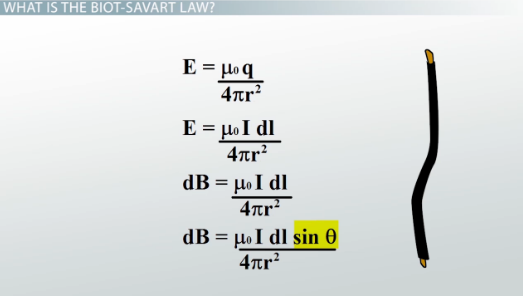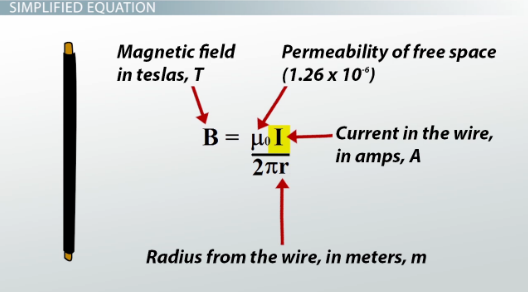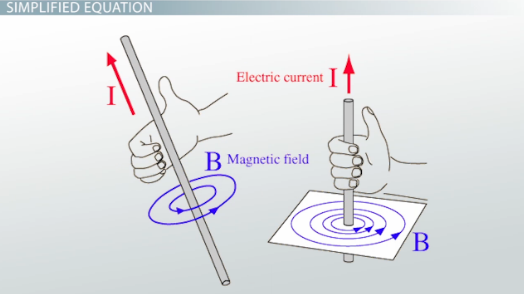# The Biot-Savart Law: Definition & Examples

An error occurred trying to load this video.

Try refreshing the page, or contact customer support.

Coming up next: Mirrors: Difference Between Plane & Spherical

### You're on a roll. Keep up the good work!

Replay
Your next lesson will play in 10 seconds
• 0:01 What Is the Biot-Savart Law?
• 1:45 Simplified Equation
• 3:25 Example Calculation
• 4:58 Lesson Summary

Want to watch this again later?

Timeline
Autoplay
Autoplay
Speed

#### Recommended Lessons and Courses for You

Lesson Transcript
Instructor: David Wood

David has taught Honors Physics, AP Physics, IB Physics and general science courses. He has a Masters in Education, and a Bachelors in Physics.

After watching this lesson you will be able to explain what the Biot-Savart Law is, and use the simplified version to solve problems. A short quiz will follow.

## What Is the Biot-Savart Law?

Electric fields and magnetic fields might seem different, but they're actually part of one larger force called the electromagnetic force. Charges that aren't moving produce electric fields. But when those charges do move, they instead create magnetic fields. For example, a magnet is only a magnet because of moving charges inside it. And charges moving in an electric wire also produce magnetic fields. If you move a compass near to an electric wire, you'll find that the compass needle changes direction.

The Biot-Savart Law is an equation that describes the magnetic field created by a current-carrying wire, and allows you to calculate its strength at various points.

To derive this law, we first take this equation for electric field. This is the full version, where we use muu-zero over 4pi instead of the electrostatic constant k. Since we're looking at a wire, we replace the charge q with I dl, which is the current in the wire, multiplied by a length element in the wire. Basically it's treating this little chunk of the wire as our charge. And we also replace the electric field E with a magnetic field element dB because a moving charge produces a magnetic field, not an electric field.

Last of all, we have to realize that a current has a direction (unlike a charge). So we need to make sure the direction of the current affects our result. We do that by adding sine of the angle between the current and the radius. That way, if the wire is curvy, we'll take that into account. And that's it - that's the Biot-Savart law.## Simplified Equation

Using the Biot-Savart Law requires calculus. That's why there's a dB and dl. Those are infinitesimal magnetic field elements and wire elements. So we'd have to integrate with respect to those elements. But we can use a simpler version of the law for a perfectly straight wire.

If we straighten out the wire and do some calculus, the law comes out as muu-zero I divided by 2pir. Or in other words, the magnetic field, B, measured in teslas is equal to the permeability of free space, muu-zero, which is always 1.26 x 10^-6, multiplied by the current going through the wire, I, measured in amps, divided by 2pi times the radius away from the wire, r, measured in meters. So this equation helps us figure out the magnetic field at a radius r from a straight wire carrying a current I.The equation gives us the magnitude of the magnetic field, but magnetic field is a vector, so what about the direction? The magnetic field created by a current-carrying wire takes the form of concentric circles. But we have to be able to figure out if those circles point clockwise or counter-clockwise (say, from above). To do that we use a right-hand rule.

I want you to give the screen a thumbs up, right now. I'm serious - give the screen a thumbs up with your right hand. It has to be with your right hand. If you point your thumb in the direction of the current for this wire, your fingers will curl in the direction of the magnetic field. They'll follow the arrows of the concentric circles. And that's how you figure out the direction.To unlock this lesson you must be a Study.com Member.

### Register to view this lesson

Are you a student or a teacher?

### Unlock Your Education

#### See for yourself why 30 million people use Study.com

##### Become a Study.com member and start learning now.
Back
What teachers are saying about Study.com

### Earning College Credit

Did you know… We have over 160 college courses that prepare you to earn credit by exam that is accepted by over 1,500 colleges and universities. You can test out of the first two years of college and save thousands off your degree. Anyone can earn credit-by-exam regardless of age or education level.